Logistic Model

Logistic Model

Logistic model was developed by Belgian mathematician Pierre Verhulst (1838) who suggested that the rate of population increase may be limited, i.e., it may depend on population density: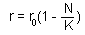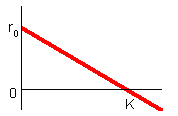At low densities (N < < K), the population growth rate is maximal and equals to ro. Parameter ro can be interpreted as population growth rate in the absence of intra-specific competition.

Population growth rate declines with population numbers, N, and reaches 0 when N = K. Parameter K is the upper limit of population growth and it is called carrying capacity. It is usually interpreted as the amount of resources expressed in the number of organisms that can be supported by these resources. If population numbers exceed K, then population growth rate becomes negative and population numbers decline. The dynamics of the population is described by the differential equation: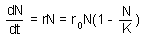which has the following solution: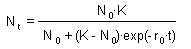Three possible model outcomes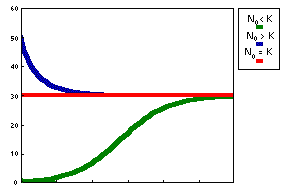Population increases and reaches a plateau (No < K). This is the logistic curve. Population decreases and reaches a plateau (No > K) Population does not change (No = K or No = 0)

Logistic model has two equilibria: N = 0 and N = K. The first equilibrium is unstable because any small deviation from this equilibrium will lead to population growth. The second equilibrium is stable because after small disturbance the population returns to this equilibrium state.

Logistic model combines two ecological processes: reproduction and competition. Both processes depend on population numbers (or density). The rate of both processes corresponds to the mass-action law with coefficients: ro for reproduction and ro/K for competition.

Interpretation of parameters of the logistic model

Parameter ro is relatively easy to interpret: this is the maximum possible rate of population growth which is the net effect of reproduction and mortality (excluding density-dependent mortality). Slowly reproducing organisms (elephants) have low ro and rapidly reproducing organisms (majority of pest insects) have high ro. The problem with the logistic model is that parameter ro controls not only population growth rate, but population decline rate (at N > K) as well. Here biological sense becomes not clear. It is not obvious that organisms with a low reproduction rate should die at the same slow rate. If reproduction is slow and mortality is fast, then the logistic model will not work.

Parameter K has biological meaning for populations with a strong interaction among individuals that controls their reproduction. For example, rodents have social structure that controls reproduction, birds have territoriality, plants compete for space and light. However, parameter K has no clear meaning for organisms whose population dynamics is determined by the balance of reproduction and mortality processes (e.g., most insect populations). In this case the equilibrium population density does not necessary correspond to the amount of resources; thus, the term "carrying capacity" becomes confusing. For example, equilibrium density may depend on mortality caused by natural enemies.

Alexei Sharov 2/03/97